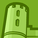# New to Qlik Sense

If you’re new to Qlik Sense, start with this Discussion Board and get up-to-speed quickly.

Announcements
LINKEDIN LIVE: Democratizing data to enhance customer-centricity. JULY 29TH REGISTER TODAY
cancel
Showing results for
Did you mean:Contributor

Hi

My ultimate aim is to calculate bad debt (outstanding for more than 31 days). Currently I managed to make it work with this (1-(Sum([Payment of loan id])/Sum([Loan amount id]))). But the user has to select the last 31 days and exclude them manually each time they want to view bad debt.

//Only({\$<[Loan Date] = {'<=\$(=Max([Loan Date])-31)'}>} (1-(Sum([Payment of loan id])/Sum([Loan amount id])))))

1-(Sum({\$<[Loan Date] = {'<=\$(=Max([Loan Date])-31)'}>}[Payment of loan id])/Sum({\$<[Loan Date] = {'<=\$(=Max([Loan Date])-31)'}>}[Loan amount id]))

So far I get a blank display

Thanks for the help

2 RepliesMaster III

Which QS version you are using, I think from Nov release onwards single quote and double quote are not considered identical, try double quote instead of apostrophe, also Loan Date needs to be date type, may be -

1-(Sum({\$<[Loan Date] = {"<=\$(=Max([Loan Date])-31)"}>}[Payment of loan id])/Sum({\$<[Loan Date] = {"<=\$(=Max([Loan Date])-31)"}>}[Loan amount id]))Contributor
Author

Hi digvijay

I tried it.. It didn't work.

1-(Sum({\$<[Loan Date] = {"<=\$(=Max([Loan Date])-31)"}>}[Payment of loan id])/Sum({\$<[Loan Date] = {"<=\$(=Max([Loan Date])-31)"}>}[Loan amount id]))Tags
Community Browser